@import url(http://www.google.com/cse/api/branding.css);Custom Search

Fountain Products
Cave Surveying
 • Cave Survey Software
Free Utilities, Tools
 • Web Image Tracker • Sports Audio Delay • Time Calibrator • Spectrum Analyzer • Used Car Evaluater • Terrain Modeling • Free Topo Maps • XEdit Text Editor • EXPL Language
Fun Stuff
• Games
• Arachnid-32
• Eight-X Solitaire
Math, Computers
• 3D Mandelbrots
• Super-Spirograph
• 6502 Memorabilia

If you like our Programs Please Leave A TIPMarching Cubes
 Marching Cubes is an algorithm for converting a 3D surface into a mesh suitable for computer graphic display. The 3D surface data can come from a variety of sources. Isosurfaces. A common source of surface data is something called an Isosurface. The prefix Iso comes from Greek and means "equal." Thus an Isosurface is a surface that follows points of equal value. As a 2D example, the lines on a weather map show lines of equal atmospheric pressure. Likewise, the lines on a topographic map show lines of equal elevation. The map to the right shows a dark-brown line 5,500 that follows points that are 5,500 feet above sea level.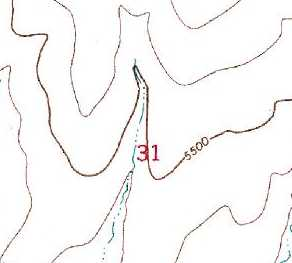In the 3D world, the Marching Cube algorithm allows you to find points of equal value and, instead of creating a line, create a surface in the form of a 3D mesh. For example, you could measure the density of tissue in a CAT-Scan and create a surface at the point the tissue becomes hard and dense. Since tissue become hard and dense at the surface of a bone, this would allow you to create a 3D model of a fractured skull to help surgeons make repairs. The image to the right shows a human skull reconstructed from CAT-Scan data using the March Cubes algorithm.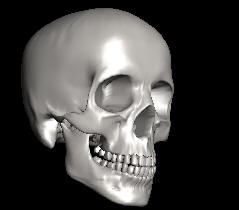Isosurface Equations. To illustrate the concept, one way of creating an isosurface is with a simple equation. For example, look at the the equation:

X * X + Y * Y + Z * Z.

It takes an X, Y and Z argument which are Cartesian Coordinates for points in 3D space and produces a value for any point in 3D space. For instance, plugging the values 1, 2 and 3 into the equation, you get a value of 14 for that point.

To use the equation to find a 3D surface, you want to find points in 3D space where the equation produces the same value. For example, here are some example points that produce values of one.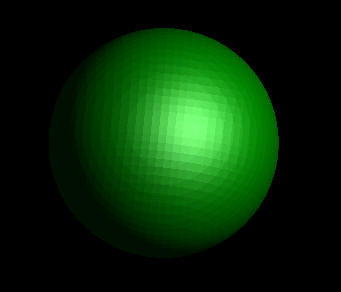X Y Z Value 1.000 0.000 0.000 = 1.000 0.707 0.707 0.000 = 1.000 0.707 0.000 0.707 = 1.000 0.000 0.707 0.707 = 1.000 0.577 0.577 0.577 = 1.000

If you examine the points where the result of the equation is one, you'll discover they form a spherical surface. The image to the above and to the right shows a spherical surface created with the equation.

Using a more complex equation produces a more complex surface. For example, using the equation 1-(Cos(X)+Cos(Y)+Cos(z)) produces a much moe complicated surface called a Schwartz Isosurface.Generating these meshes is actually more difficult than it seems. To find points that are on the surface, you have to find a large number of points whose values are all one. This means testing millions of points, most of which are nowhere near the surface. The solution is to use a process called Marching Cubes. The Marching Cubes algorithm simplifies this process by dividing the 3D space into cubic boxes. The program only tests the points at the corners of the boxes to see if the surface passes through the box. If the surface passes through box, the exactly location of the surface is calculated by interpolation.For example, using the sphere equation we can calculate where the surface of the sphere intersects the cube. In example to the right, the black numbers represent the corner coordinates of the one side of the cube. The red numbers show the sphere-equation value for those coordinates. As you can see, the surface of the sphere, (as represented by the 1-value,) falls somewhere along the top and right sides of the box. By interpolating, we can find the exact location.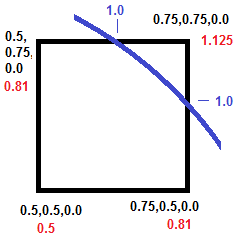Since the ultimate goal is to create a 3D mesh, we have to find the places where the surface intersects each cubes and create triangles that correspond to the planes of intersections. Since there are only a limited number of ways these planes of intersections can occur, we can rapidly determine where the triangles need to be placed. Once we where the triangles need to places we can determine the exact location interpolating the intersection points just like we did with the 2D example above. The Drawings to the right show all the possible combinations. Corners with an orange dot are inside the surface. Points without a dot are outside.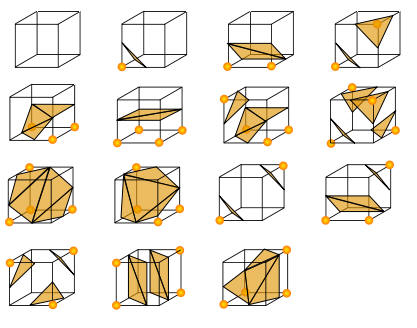The triangles shown are based on the pattern of dots that are inside and outside the surface. For more details on the Marching Cubes algorithm, click here.
 Resolution. The resolution of the mesh generated by the Marching Cubes algorithm is controlled by the number of cubes. The more cubes, the smaller each cube and the more detail the algorithm captures. On the other hand, the number of cells goes up by the cube of the number of grid lines. That means a 5 x 5 x 5 grid will have 125 cells, a 10 x 10 x 10  grid will have 1,000 cells and a 100 x 100 x 100 cell grid will have 1 million cells. As a result, the processing time also goes up by the number cube of the grid lines. For example, a 50 x 50 x 50 grid can be process in one second, 100 x 100 x 100  takes 5 seconds and a 200 x 200 x 200 takes 36 seconds.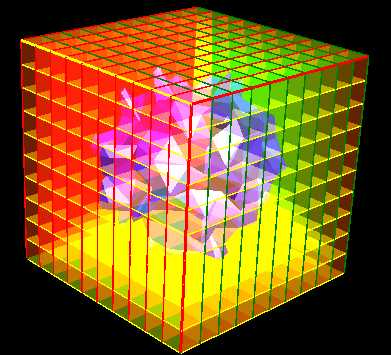To control the resolution, Mandelbrot 3D allows you to set the number of grid lines. The "Grid" option shown to the right sets the number of grid lines. Range. The Maximum and Minimum range values are the 3D coordinates of the minimum and maximum range covered by the Marching Cubes algorithm. Setting the smaller values allows you to focus on smaller portions of the surface.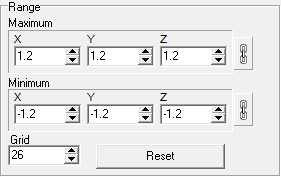` `

 Contact UsCustom Search